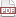### Another C macro trick: parenthesized arguments

09 August 13. [link]PDF version

I just realized another thing about C macros. On the one hand, I feel bad that I didn't realize this when I was writing 21st Century C, a book half-filled with tips like these; on the other hand, it feels criminal that of the dozens of textbooks I read about C before writing mine, none of them mentioned this.

Rest assured that this will be included in the next revision of 21st Century C. Part of the joy of having a textbook here in the modern day, especially with a tech-oriented publisher, is that we can send a new camera-ready PDF to the printer at whim, and it'll be a printed book within a few weeks. Every error anybody on the Internet said was in the book has at this point been addressed, which is pretty nice.

###### Parens in macros

First, if you're not familiar with variadic macros—because there are still C textbooks sold today that fail to mention them—have a look at either this earlier entry or the discussion in 21st Century C.

The problem is when you want to merge two variadic macros or functions. For example, say that you've decided that your program should emit errors in two ways: print a more human-friendly message to screen and print a machine-readable error code to a log (I'll just use stderr). It would be nice to have one function that takes in printf-style arguments to both output functions, but then how would the compiler know when one set of arguments ends and the next begins?

We can group arguments the way we always do: parens. From both the C99 and C11 standards:

§6.10.3(11): The individual arguments within the list are separated by comma preprocessing tokens, but comma preprocessing tokens between matching inner parentheses do not separate arguments.

And thus, here is a workable example to print two error messages at once:

#define fileprintf(...) fprintf(stderr, __VA_ARGS__)
#define doubleprintf(human, machine) do {printf human; fileprintf machine;} while(0)

//usage:
if (x < 0) doubleprintf(("x is less than zero (value: %g)\n", x), ("NEGVAL: x=%g\n", x))


The macro will expand to:

do {printf ("x is less than zero (value: %g)\n", x); fileprintf ("NEGVAL: x=%g\n", x);} while(0);


I added that fileprintf macro to provide consistency across the two statements. Without it, you'd need the human printf arguments in parens and the log printf arguments not in parens:

#define doubleprintf(human, ...) do {printf human; fprintf (stderr, __VA_ARGS__);} while(0)

//and so:
if (x < 0) doubleprintf(("x is less than zero (value: %g)\n", x), "NEGVAL: x=%g\n", x)


I don't like this from the user interface (UI) perspective, because symmetric things should look symmetric.

What if users forget the parens entirely? It won't compile: there isn't much that you can put after printf besides an open paren that won't give you a cryptic error message. On the one hand, you get a cryptic error message; on the other, there's no way to accidentally forget the parens and ship wrong code into production.

Variadic macros were a really nice addition to the language, and are significantly safer than the raw variadic function form, which is so error-prone that it treads in security-flaw territory. Throw in a few extra parens, and you have something that really extends the utility of the form, even if it isn't the traditional C UI.

###### Example two: allocate and fill a data set

The apop_data_alloc macro+function takes in zero through three arguments, depending on whether you want a blank data set, a vector, a matrix, or a vector-matrix pair. The apop_data_fill macro+function takes in enough arguments to fill a matrix. I get the number of arguments from the dimensions listed in the already-allocated matrix.

Typical usage:

apop_data *d = apop_data_alloc(2,2);
apop_data_fill(d,
1, 0,
0, 1);
//or more briefly:

apop_data *d = apop_data_fill(apop_data_alloc(2,2),
1, 0,
0, 1);


It would be nice to have a macro that provides dimensions for the alloc function and data for the fill function, and we can do so using the paren trick:

#define alloc_fill(dims, ... ) apop_data_fill(apop_data_alloc dims, __VA_ARGS__);

apop_data *d = alloc_fill((2,2),
1, 0,
0, 1);


Given that it is not uncommon to have little fixed-contents vectors and matrices all over a program, having a simplified UI for creating them can really improve the flow.

###### Example 3: a times table

This final example will generate a product table: given two lists $R$ and $C$, each cell $(i, j)$ will hold the product $R_i\cdot C_j$.


#include <gsl/gsl_matrix.h>
#include <math.h>
#include <apop.h> //just for apop_matrix_print

#define make_a_list(...) (double[]){__VA_ARGS__, NAN}

#define matrix_cross(list1, list2) matrix_cross_base(make_a_list list1, make_a_list list2)

gsl_matrix *matrix_cross_base(double *list1, double *list2){
int count1 = 0, count2 = 0;
while(!isnan(list1[count1])) count1++;
while(!isnan(list2[count2])) count2++;
if (!count1 || !count2) return NULL;

gsl_matrix *out = gsl_matrix_alloc(count1, count2);
for (int i=0; i< count1; i++)
for (int j=0; j< count2; j++)
gsl_matrix_set(out, i, j, list1[i]*list2[j]);
return out;
}

int main(){
gsl_matrix *m = matrix_cross((1,2,4,8), (5, 11, 15));
apop_matrix_print(m);

printf("\n\n");
apop_matrix_print( matrix_cross((17, 19, 23), (1,2,3,5,7,11,13)) );

//create and fill a column vector
printf("\n\n");
apop_matrix_print( matrix_cross((1,2,3,5,7,11,13), (1)) );
}


Next time, more about the apop_data struct, and a whole lot of data manipulation functions.